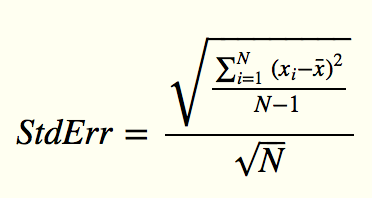# Info dialog box ANOVA statistics

3 posts / 0 new
WBYInfo dialog box ANOVA statistics

The statistics pane of the info dialog contains the following statement:

"Confidence Interval: Mean error bars denote 95% Confidence Interval (twice Standard Error)"

My question is which part of the statement is true, 95% confidence interval or twice Standard Error? If the sample size in bins is large enough then 2 sigma is ~95%. However, often bins contain very small samples and the "T" distribution applies applies rather than a Gaussian distribution to account for the increasing likelihood that the sample is not representative of the population as the sample size decreases (sampling error). For sample sizes of 30 or more the two distributions are frequently taken to be the same. However, when you have a sample size of 10 95% confidence interval (2 tailed test) is about 2.3 sigma and at a sample size of 5 it is almost 2.8 sigma.

I suspect the error bars are 2-sigma but I think the statement needs to be modified.t-table.pdf
David BennCI: std error

It's 2 x standard error. Here’s the calculation used for standard error in VStar by the way:where N is the number of observations.

So, given your reasonable argument, should we create a ticket to remove the 95% CI note from the Info dialog?

Comments from others is welcome. I'm not a statistician.

Davidstderr.png